# Figures for the MBR Manuscript

This vignette produces the graphs included in the initial MBR manuscript.

## Figure 1: Cartesian Rolling - 2005 Version

Figure 1: Raw monthly birth rates (General Fertility Rates; GFRs) for Oklahoma County, 1990-1999, plotted in a linear plot; the “bombing effect” is located ten months after the Oklahoma City bombing.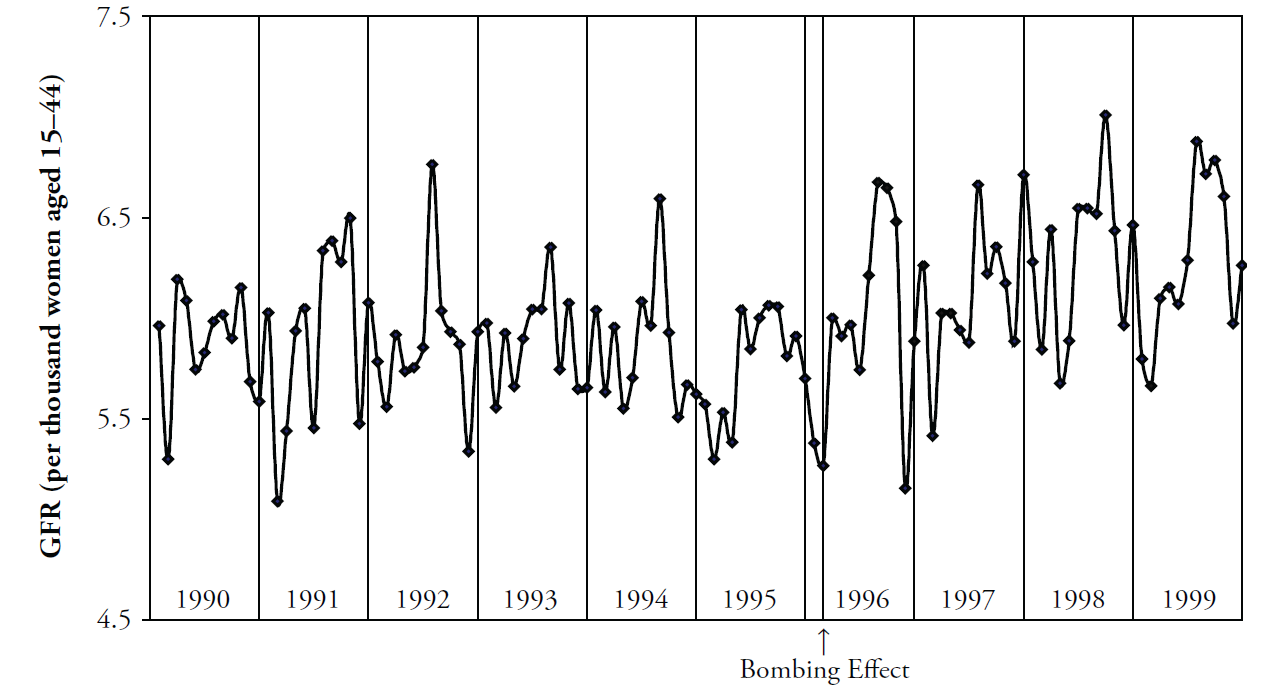## Figure 2: Cartesian Rolling - 2014 Version

Smoothed monthly birth rates (General Fertility Rates; GFRs) for Oklahoma County, 1990-1999, plotted in a linear plot. The top plot shows the connected raw data with a February smoother; the middle plot shows smoothing with a 12-month moving average, blue/green line, superimposed on a February smoother, red line); the bottom plot shows the smoothers and confidence bands, which are H-spreads defined using the distribution of GFRs for the given month and 11 previous months.

First, some R packages are loaded, and some variables and functions are defined.

``````change_month <- base::as.Date("1996-02-15") #as.Date("1995-04-19") + lubridate::weeks(39) = "1996-01-17"
set.seed(444) # So bootstrap won't trigger a git diff

vp_layout <- function(x, y) {
grid::viewport(layout.pos.row = x, layout.pos.col = y)
}
base::range(scores) # A new function isn't necessary.  It's defined in order to be consistent.
}
stats::quantile(x = scores, probs = c(.25, .75))
}
base::mean(scores) + base::c(-1, 1) * stats::sd(scores) / base::sqrt(base::sum(!base::is.na(scores)))
}
boot_spread <- function(scores, conf = .68) {
plugin <- function(d, i) {
base::mean(d[i])
}

distribution <- boot::boot(data = scores, plugin, R = 99) # 999 for the publication
ci <- boot::boot.ci(distribution, type = c("bca"), conf = conf)
ci\$bca[4:5] # The fourth & fifth elements correspond to the lower & upper bound.
}

dark_theme <- ggplot2::theme(
axis.title          = ggplot2::element_text(color = "gray30", size = 9),
axis.text.x         = ggplot2::element_text(color = "gray30", hjust = 0),
axis.text.y         = ggplot2::element_text(color = "gray30"),
axis.ticks          = ggplot2::element_blank(),
# panel.grid.minor.y  = element_line(color = "gray95", linewidth = .1),
# panel.grid.major    = element_line(color = "gray90", linewidth = .1),
panel.spacing       = grid::unit(c(0, 0, 0, 0), "cm"),
plot.margin         = grid::unit(c(0, 0, 0, 0), "cm")
)
# qplot(mtcars\$hp) + dark_theme

light_theme <-
dark_theme +
ggplot2::theme(
axis.title          = ggplot2::element_text(color = "gray80", size = 9),
axis.text.x         = ggplot2::element_text(color = "gray80", hjust = 0),
axis.text.y         = ggplot2::element_text(color = "gray80"),
panel.grid.minor.y  = ggplot2::element_line(color = "gray99", linewidth = .1),
panel.grid.major    = ggplot2::element_line(color = "gray95", linewidth = .1)
)
date_sequence <-
base::seq.Date(
from = base::as.Date("1990-01-01"),
to   = base::as.Date("1999-01-01"),
by   = "years"
)
x_scale <-
ggplot2::scale_x_date(
breaks = date_sequence,
labels = scales::date_format("%Y")
)
# This keeps things proportional down the three frames.
x_scale_blank <-
ggplot2::scale_x_date(
breaks = date_sequence,
labels = NULL
)``````

### Individual Components

Here is the basic linear rolling graph. It doesn’t require much specification, and will work with a wide range of appropriate datasets. This first (unpublished) graph displays all components.

``````# Uncomment the next two lines to use the version built into the package.  By default, it uses the
# CSV to promote reproducible research, since the CSV format is more open and accessible to more software.

ds_linear_all <-
county_month_birth_rate_2005_version |>
tibble::as_tibble()

ds_linear_okc <-
ds_linear_all |>
dplyr::filter(county_name == "oklahoma") |>
augment_year_data_with_month_resolution(date_name = "date")

portfolio_cartesian <-
annotate_data(
ds_linear_okc,
dv_name         = "birth_rate",
center_function = stats::median,
)

cartesian_rolling(
ds_linear           = portfolio_cartesian\$ds_linear,
x_name              = "date",
y_name              = "birth_rate",
stage_id_name       = "stage_id",
change_points       = change_month,
change_point_labels = "Bombing Effect"
)``````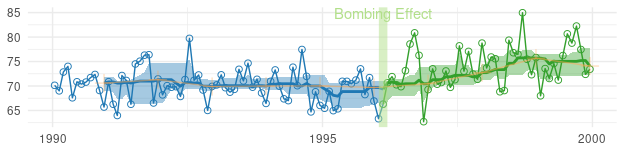The version for the manuscript was tweaked to take advantage of certain features of the dataset. This is what it looks like when all three stylized panels are combined.

``````top_panel <-
Wats::cartesian_rolling(
ds_linear             = portfolio_cartesian\$ds_linear,
x_name                = "date",
y_name                = "birth_rate",
stage_id_name         = "stage_id",
change_points         = change_month,
y_title               = "General Fertility Rate",
change_point_labels   = "Bombing Effect",
draw_rolling_band     = FALSE,
draw_rolling_line     = FALSE
)

middle_panel <-
Wats::cartesian_rolling(
ds_linear             = portfolio_cartesian\$ds_linear,
x_name                = "date",
y_name                = "birth_rate",
stage_id_name         = "stage_id",
change_points         = change_month,
y_title               = "General Fertility Rate",
change_point_labels   = "",
draw_rolling_band     = FALSE,
draw_jagged_line      = FALSE
)

bottom_panel <-
Wats::cartesian_rolling(
ds_linear             = portfolio_cartesian\$ds_linear,
x_name                = "date",
y_name                = "birth_rate",
stage_id_name         = "stage_id",
change_points         = change_month,
y_title               = "General Fertility Rate",
change_point_labels   = "",
# draw_rolling_band   = FALSE,
draw_jagged_line      = FALSE
)

top_panel    <- top_panel    + x_scale       + dark_theme
middle_panel <- middle_panel + x_scale       + dark_theme
bottom_panel <- bottom_panel + x_scale_blank + dark_theme

grid::grid.newpage()
grid::pushViewport(grid::viewport(layout = grid::grid.layout(3,1)))
print(top_panel   , vp = vp_layout(1, 1))
print(middle_panel, vp = vp_layout(2, 1))
print(bottom_panel, vp = vp_layout(3, 1))
grid::popViewport()``````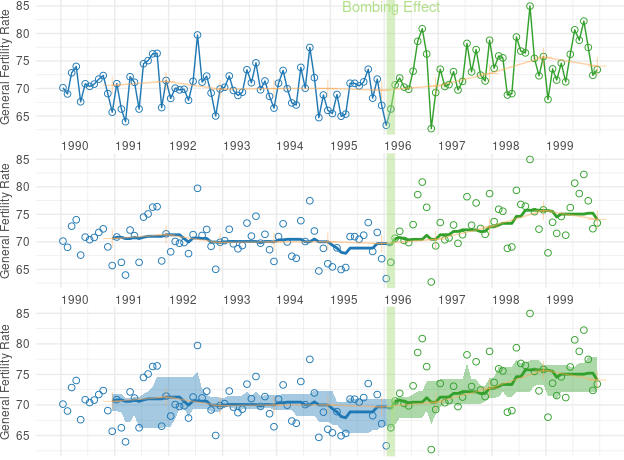## Figure 4: Cartesian Periodic

Cartesian plot of the GFR time series data in Oklahoma County, with H-spread Bands superimposed.

``````cartesian_periodic <-
Wats::cartesian_periodic(
portfolio_cartesian\$ds_linear,
portfolio_cartesian\$ds_periodic,
x_name                  = "date",
y_name                  = "birth_rate",
stage_id_name           = "stage_id",
change_points           = change_month,
change_point_labels     = "Bombing Effect",
y_title                 = "General Fertility Rate",
draw_periodic_band      = TRUE #The only difference from the simple linear graph above
)
print(cartesian_periodic)``````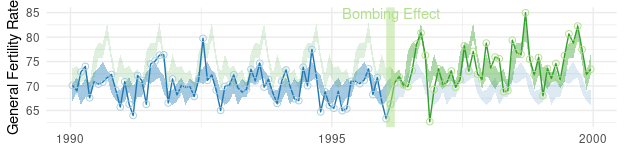``````cartesian_periodic <- cartesian_periodic + x_scale + dark_theme
print(cartesian_periodic)``````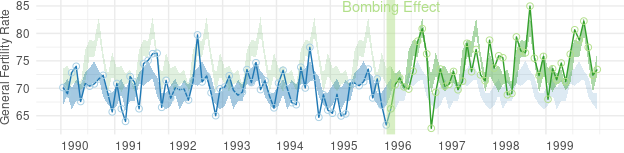## Figure 5: Polar Periodic

Wrap Around Time Series (WATS Plot) of the Oklahoma City GFR data, 1990-1999.

``````portfolio_polar <-
polarize_cartesian(
ds_linear                       = portfolio_cartesian\$ds_linear,
ds_stage_cycle                  = portfolio_cartesian\$ds_stage_cycle,
y_name                          = "birth_rate",
stage_id_name                   = "stage_id",
plotted_point_count_per_cycle   = 7200
)

grid::grid.newpage()
polar_periodic(
ds_linear             = portfolio_polar\$ds_observed_polar,
ds_stage_cycle        = portfolio_polar\$ds_stage_cycle_polar,
stage_id_name         = "stage_id",
draw_periodic_band    = FALSE,
draw_stage_labels     = TRUE,
cardinal_labels       = c("Jan1", "Apr1", "July1", "Oct1")
)``````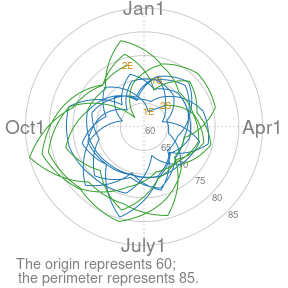## Figure 6: WATS and Cartesian

Wrap Around Time Series (WATS Plot) of the Oklahoma City GFR data, 1990-1999.

``````portfolio_polar <-
Wats::polarize_cartesian(
ds_linear                     = portfolio_cartesian\$ds_linear,
ds_stage_cycle                = portfolio_cartesian\$ds_stage_cycle,
y_name                        = "birth_rate",
stage_id_name                 = "stage_id",
plotted_point_count_per_cycle = 7200
)

grid::grid.newpage()
grid::pushViewport(grid::viewport(
layout = grid::grid.layout(
nrow    = 2,
ncol    = 2,
respect = TRUE,
widths  = grid::unit(c(1,  1), c("null", "null")),
heights = grid::unit(c(1, .5), c("null", "null"))
),
gp = grid::gpar(cex = 1, fill = NA)
))

## Create top left panel
grid::pushViewport(grid::viewport(layout.pos.col = 1, layout.pos.row = 1))
top_left_panel <-
Wats::polar_periodic(
ds_linear             = portfolio_polar\$ds_observed_polar,
ds_stage_cycle_polar  = portfolio_polar\$ds_stage_cycle_polar,
stage_id_name         = "stage_id", #graph_ceiling = 7,
cardinal_labels       = c("Jan1", "Apr1", "July1", "Oct1")
)
grid::upViewport()

## Create top right panel
grid::pushViewport(grid::viewport(layout.pos.col = 2, layout.pos.row = 1))
top_right_panel <-
Wats::polar_periodic(
ds_linear             = portfolio_polar\$ds_observed_polar,
ds_stage_cycle_polar  = portfolio_polar\$ds_stage_cycle_polar,
stage_id_name         = "stage_id", #graph_ceiling = 7,
draw_observed_line    = FALSE,
cardinal_labels       = c("Jan1", "Apr1", "July1", "Oct1"),
origin_label          = NULL
)
grid::upViewport()

## Create bottom panel
grid::pushViewport(grid::viewport(layout.pos.col = 1:2, layout.pos.row = 2, gp = grid::gpar(cex = 1)))
print(cartesian_periodic, vp = vp_layout(x = 1:2, y = 2)) # Print across both columns of the bottom row.
grid::upViewport()``````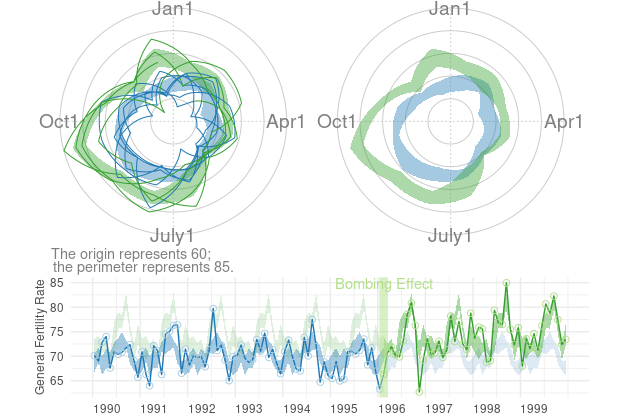## Figure 7: County Comparison

This figure compares Oklahoma County against the (other) largest urban counties.

``````# ds_linear_all <- Wats::augment_year_data_with_month_resolution(ds_linear = county_month_birth_rate_2005_version, date_name="date")

# Identify the average size of the fecund population
ds_linear_all |>
dplyr::group_by(county_name) |>
dplyr::summarize(
Mean = base::mean(fecund_population)
) |>
dplyr::ungroup()``````
``````# A tibble: 12 × 2
county_name     Mean
<chr>          <dbl>
2 cleveland     48865.
3 comanche      26268.
4 creek         13402.
5 logan          7065.
6 mcclain        5434.
7 oklahoma     146882.
8 osage          8529.
9 pottawatomie  13604.
10 rogers        13383.
11 tulsa        123783.
12 wagoner       11580.``````
``````graph_row_comparison <- function(
row_label       = "",
.county_name    = "oklahoma",
change_month    = as.Date("1996-02-15")
) {
ds_linear <-
ds_linear_all |>
dplyr::filter(county_name == .county_name) |>
Wats::augment_year_data_with_month_resolution(date_name = "date")

portfolio_cartesian <-
ds_linear |>
Wats::annotate_data(
dv_name         = "birth_rate",
center_function = stats::median,
)

portfolio_polar <-
portfolio_cartesian\$ds_linear |>
Wats::polarize_cartesian(
ds_stage_cycle                = portfolio_cartesian\$ds_stage_cycle,
y_name                        = "birth_rate",
stage_id_name                 = "stage_id",
plotted_point_count_per_cycle = 7200
)

cartesian_periodic <-
portfolio_cartesian\$ds_linear |>
Wats::cartesian_periodic(
portfolio_cartesian\$ds_periodic,
x_name                            = "date",
y_name                            = "birth_rate",
stage_id_name                     = "stage_id",
change_points                     = change_month,
change_point_labels               = ""
)

grid::pushViewport(grid::viewport(
layout =
grid::grid.layout(
nrow    = 1,
ncol    = 3,
respect = FALSE,
widths  = grid::unit(c(1.5, 1, 3), c("line", "null", "null"))
),
gp = grid::gpar(cex = 1, fill = NA)
))

grid::pushViewport(grid::viewport(layout.pos.col = 1))
grid::grid.rect(gp = grid::gpar(fill = "gray90", col = NA))
grid::grid.text(row_label, rot = 90)
grid::popViewport()

grid::pushViewport(grid::viewport(layout.pos.col = 2))
Wats::polar_periodic(
ds_linear               = portfolio_polar\$ds_observed_polar,
ds_stage_cycle_polar    = portfolio_polar\$ds_stage_cycle_polar,
draw_observed_line      = FALSE,
stage_id_name           = "stage_id",
origin_label            = NULL,
plot_margins            = c(0, 0, 0, 0)
)
grid::popViewport()

grid::pushViewport(grid::viewport(layout.pos.col = 3))
print(cartesian_periodic + x_scale + light_theme, vp = vp_layout(x = 1, y = 1))
grid::popViewport()
grid::popViewport() #Finish the row
}

county_names  <- c("Comanche", "Cleveland", "Oklahoma", "Tulsa", "Rogers")
counties      <- tolower(county_names)

grid::grid.newpage()
grid::pushViewport(grid::viewport(
layout  = grid::grid.layout(nrow = length(counties), ncol = 1),
gp      = grid::gpar(cex = 1, fill = NA)
))

for (i in base::seq_along(counties)) {
grid::pushViewport(grid::viewport(layout.pos.row = i))
graph_row_comparison(.county_name = counties[i], row_label = county_names[i])
grid::popViewport()
}
grid::popViewport()``````